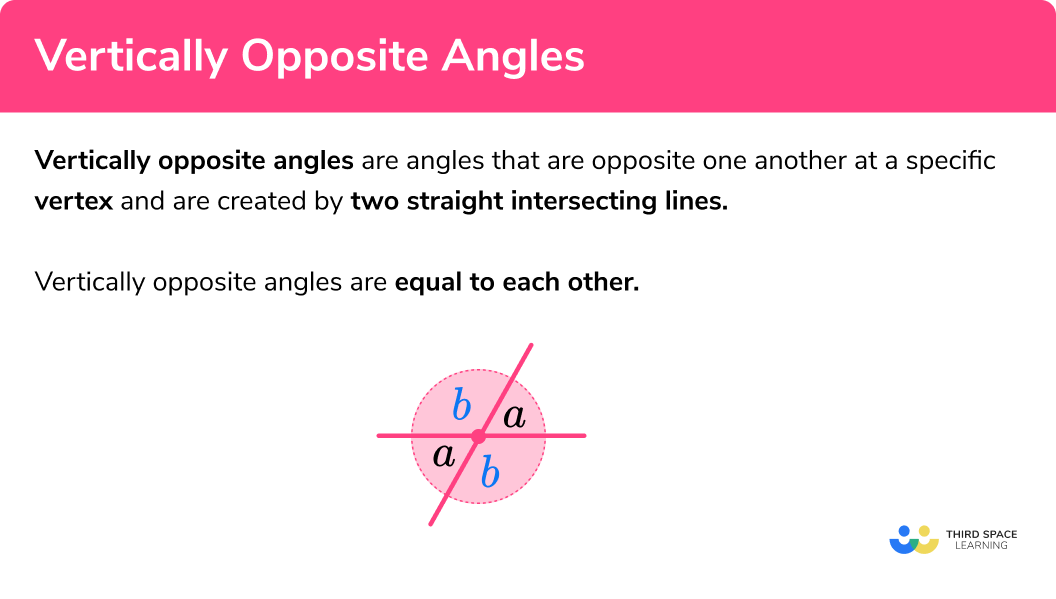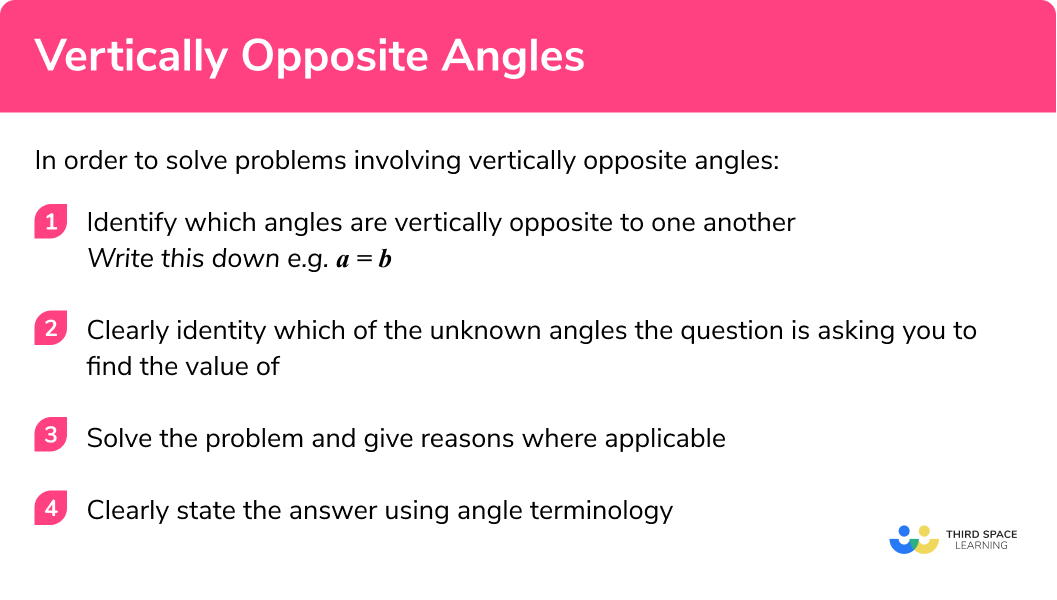GCSE Maths Geometry and Measure Angles Angle Rules

Vertically Opposite Angles

# Vertically Opposite Angles

Here we will learn about vertically opposite angles including how to find missing angles which are vertically opposite each other at the same vertex.

There are also angles in polygons worksheets based on Edexcel, AQA and OCR GCSE exam style questions, along with further guidance on where to go next if you’re still stuck.

## What are vertically opposite angles?

Vertically opposite angles are angles that are opposite one another at a specific vertex and are created by two straight intersecting lines

Vertically opposite angles are equal to each other.

These are sometimes called vertical angles.

Here the two angles labelled ‘a’ are equal to one another because they are ‘vertically opposite’ at the same vertex. This also applies to the angles labelled ‘b’

You can try out the above rule by drawing two crossing lines and measuring the angles opposite to one another.
You will also notice that angle ‘a’ and ‘b’ lie on a straight line and are therefore equal to 180 degrees when added together. See below:

Note because the sum of angles ‘a’ and ‘b’ are 180º we can call them supplementary angles.

Before we start looking at specific examples it is important we are familiar with some key words, terminology and symbols required for this topic.

### What are vertically opposite angles?### Keywords

• Angle: defined as the amount of turn around a common vertex.
• Vertex: the point created by two line segments meeting (plural is vertices)

• How to label an angle:

We normally label angles in two main ways:

1By giving the angle a ‘name’ which is normally a lower case letter such as a, x or y or the greek letter ϴ (theta).

2By referring to the angle as the three letters that define the angle. The middle letter refers to the vertex at which the angle is e.g. see the diagram for the angle we call ABC:

• Angles on a straight line equal 180º:

Angles on one part of a straight line always add up to 180º. See the diagram for an example where angles a and b are equal to 180º:

However see the next diagram for an example of where a and b do not equal 180º because they are not on one single part of a straight line, i.e. they do not share a vertex and are not adjacent to one another.

Note – you can try out the above rule by drawing out the above diagrams and measuring the angles using a protractor.

• Angles around a point equal 360º:

Angles around a point will always equal 360º. See the diagram for an example where angles a, b and c are equal to 360º

• Supplementary and Complementary Angles:

Two angles are supplementary when they add up to 180º, they do not have to be next to each other (see below on ).

Two angles are complementary  when they add up to 90º, they do not have to be next to each other (see the diagram).

## How to solve vertically opposite angles problems

In order to solve problems involving angles you should follow these steps:

1. Identify which angles are vertically opposite to one another.
Write this down e.g. a = b.
2. Clearly identity which of the unknown angles the question is asking you to find the value of.
3. Solve the problem and give reasons where applicable.
4. Clearly state the answer using angle terminology.

### How to solve vertically opposite angles problems.## Vertically opposite angles examples

### Example 1: two angles that are vertically opposite

Find the value of angle x.

1. Identify which angles are vertically opposite to one another.

The angle labelled x and the angle with value 117º are vertically opposite to one another at the point where the two lines cross.

2 Clearly identify which of the unknown angles the question is asking you to find the value of.

The angle labelled x.

3 Solve the problem and give reasons where applicable.

x = 117 because the angles are vertically opposite to one another.

4 Clearly state the answer using angle terminology.

x = 117º

### Example 2: two angles that are vertically opposite

Find the values of angles x and y.

The angle labelled x and the angle with value 93º are vertically opposite to one another.

The angles labelled x and y.

You will notice that x and y are not vertically opposite one another.

x = 93 because the angles are vertically opposite to one another.

\begin{aligned} x+y &= 180 \hspace{3cm} \text{because they are on a straight line at the same vertex}\\\\ 93+y &= 180 \hspace{3cm} \text{subtract 93 from each side} \\\\ y&=87 \end{aligned}

x = 93º, y = 87º

### Example 3: finding all the angles around a point with vertically opposite angles

Find the values of the angles labelled a, b and c.

The angle labelled a and the angle with value 22º are vertically opposite to one another.

The angle labelled b and the angle labelled c are vertically opposite to one another.

The angles labelled a, b and c.

a = 22 because the angles are vertically opposite to one another.

\begin{aligned} a+b &= 180 \hspace{2cm} & \text{because they are on a straight line at the same vertex}\\\\ 22+b &= 180 & \text{subtract 22 from each side} \\\\ b&=158 \end{aligned}

c = b because the angles are vertically opposite to one another.

c = 158

a = 22º, b = 158º, c = 158º

### Example 4: vertically opposite angles with algebra

Using vertically opposite angles find the value of x.

The angle labelled ‘x + 10’ is vertically opposite an angle the other side of the vertex (see below).

The angle labelled ‘x + 120’ is vertically opposite an angle the other side of the vertex (see below.)

We are not being asked to find an angle we are being asked to find the value of x.

The four angles total 360º because they are all around a vertex. Therefore:

\begin{aligned} x+10+x+120+x+10+x+120 &= 360 \hspace{.5cm} & \text{simplify the equation}\\\\ 4x+260 &= 360 & \text{subtract 260 from each side} \\\\ 4x&=100 & \text{divide each side by 4} \\\\ x&=25 \end{aligned}

x = 25º

### Example 5: problem solving

In the diagram below ACD is a straight line:

AB = AC

Find the value of angle BAC.

The angle of size 80º is vertically opposite angle BCA.

Find angle BAC at the top vertex of the triangle.

ACB = 80º because the angles are vertically opposite one another.

ABC = ACB because triangle ABC is a isosceles triangle, therefore ABC = 80º.

Angle BAC + ABC + ACB = 180 because they are three interior angles of the triangle.

\begin{aligned} B A C+80+80&=180 \\\\ BAC+160&=180 \\\\ BAC&=20 \end{aligned}

Angle BAC = 20º

### Example 6: worded problem

Two angles with values of x + 30 and 4x − 30 are vertically opposite one another.

Prove the two angles are both 50º.

The angles labelled x + 30 and 4x − 30  vertically opposite to one another meaning they are equal to one another.

You are being asked to prove the size of each angle is 50º . This means show all your working to reach this conclusion.

\begin{aligned} x+30&=4x-30 \hspace{.25cm} & \text{because the angles are vertically opposite to one another}\\\\ x+60&=4x & \text{add 30 to both sides} \\\\ 60 &=3x & \text{subtract x from each side} \\\\ 20 &=x & \text{divide each side by 3} \end{aligned}

Therefore the size of the two angles can be found by substitution x = 20 into each angle:

Angle 1:

\begin{aligned} x+30&=20+30\\\\ x&=50^{\circ} \end{aligned}

Angle 2:

\begin{aligned} 4x-30&=4(20)-30\\\\ x&=50^{\circ} \end{aligned}

Therefore both angles are 50º.

### Common misconceptions

• Incorrectly labelling angles which are vertically opposite one another
• Misuse of the ‘straight line’ rule where angles do not share a vertex
• Finding the incorrect angle due to misunderstanding the terminology

Vertically opposite angles is part of our series of lessons to support revision on angle rules. You may find it helpful to start with the main angle rules lesson for a summary of what to expect, or use the step by step guides below for further detail on individual topics. Other lessons in this series include:

### Practice vertically opposite angles questions

1.  Find the value of the angle labelled x :x=113x=23x=67x=22Angle x is vertically opposite the given angle of 67^{\circ}  so it is the same.

2.  Find the value of the angle labelled x :x=146x=56x=34x=214Angle x is vertically opposite the given angle of 146^{\circ} so it is the same.

3.  Find the value of the angle labelled x :x=72x=86x=82x=98Angle x is vertically opposite the given angle of 98^{\circ} so it is the same.

4.  Find the value of the angle labelled x and  y :x=68,  y=68x=112,  y=112x=112,  y=68x=68,  y=112Angle x is vertically opposite the given angle of 112^{\circ} so it is the same.

Angle x and angle y lie on a straight line so they must add up to 180 .

5. Two angles with values of 2x and 50^{\circ} are vertically opposite one another. Find the value of x .

x=50x=25x=100x=75Angle 2x is vertically opposite the given angle of 50^{\circ} so it is the same.

To solve for x , we divide 50 by 2 .

6. Two angles with values of 6x+10 and 10x-70 are vertically opposite one another. Find the value of x

x=20x=40x=70x=10Angle 6x + 10 is vertically opposite angle of 10x  −  70 so they are the same.

Solving the equation, 6x + 10 = 10x  −  70 , leads to the correct value for x .

### Vertically opposite angles GCSE exam questions

1.  Find the size of angles a and b .(2 marks)

a = 142^{\circ} (because vertically opposite angles are equal)

(1)

b: 180 − 142 = 38^{\circ} (because angles on a straight line add to 180)

(1)

a = 142^{\circ}, b = 38^{\circ}

2.(a)  Write an equation involving x.

(b)  Use your equation to find the size of the angles.

(4 marks)

(a)

2x + 14 = 3x  −  5

(1)

(b)

14 = x  −  5

(1)

x = 19

(1)

Angles:

2 \times 19 + 14 = 52

= 52^{\circ}

(1)

3.  Prove that triangle ABC  is a right angle triangle(3 marks)

Angle  ACB = 24^{\circ} since they are vertically opposite

(1)

66 + 24 = 90

(1)

180  −  90 = 90  (angles in a triangle add up to 180 ),

so angle BAC is 90^{\circ} and this is a right angle triangle.

(1)

## Learning checklist

You have now learned how to:

• Use conventional terms and notation for angles
• Apply the properties of vertically opposite angles
• Apply angle facts and properties to solve problems

## Still stuck?

Prepare your KS4 students for maths GCSEs success with Third Space Learning. Weekly online one to one GCSE maths revision lessons delivered by expert maths tutors.

Find out more about our GCSE maths tuition programme.